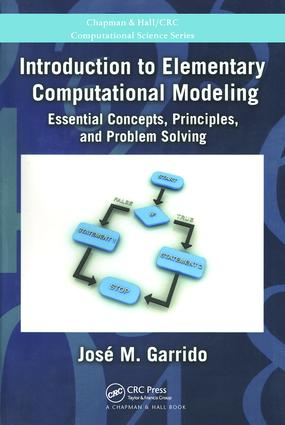# Introduction to Elementary Computational Modeling

## Essential Concepts, Principles, and Problem Solving, 1st Edition

Chapman and Hall/CRC

329 pages | 100 B/W Illus.

Paperback: 9781439867396
pub: 2011-10-26
SAVE ~\$21.00
\$105.00
\$84.00
x
eBook (VitalSource) : 9780429087042
pub: 2011-10-26
from \$50.00

FREE Standard Shipping!

### Description

With an emphasis on problem solving, this book introduces the basic principles and fundamental concepts of computational modeling. It emphasizes reasoning and conceptualizing problems, the elementary mathematical modeling, and the implementation using computing concepts and principles. Examples are included that demonstrate the computation and visualization of the implemented models.

The author provides case studies, along with an overview of computational models and their development. The first part of the text presents the basic concepts of models and techniques for designing and implementing problem solutions. It applies standard pseudo-code constructs and flowcharts for designing models. The second part covers model implementation with basic programming constructs using MATLAB®, Octave, and FreeMat.

Aimed at beginning students in computer science, mathematics, statistics, and engineering, Introduction to Elementary Computational Modeling: Essential Concepts, Principles, and Problem Solving focuses on fundamentals, helping the next generation of scientists and engineers hone their problem solving skills.

### Reviews

… offers a solid first step into scientific and technical computing for those just getting started. … Through simple examples that are both easy to conceptualize and straightforward to express mathematically (something that isn’t trivial to achieve), Garrido methodically guides readers from problem statement and abstraction through algorithm design and basic programming. His approach offers those beginning in a scientific or technical discipline something unique; a simultaneous introduction to programming and computational thinking that is very relevant to the practical application of computing many readers will experience later in their academic training, or early in their professional career.

—John West, SIGHPC Connect Newsletter, Vol. 1, June 2012

The fundamental elements of computational modeling are introduced in this nice book. … targets undergraduate students in computer science and allied subjects such as statistics, mathematics, and engineering. The book should also be well received by teachers and other scientists associated with these disciplines.

Computing Reviews May 2012

Each chapter includes a Summary, Key Terms, and Exercises

Understanding Problem Solving

Understanding Word Problems

Introduction

Nouns and Verbs

Problem Decomposition

The Language of Computational Problems

Objects

Problems with Many Numbers

Problem Solving and Computing

Introduction

Programs

Data Definitions

Programming Languages

Interpretation of Commands with MATLABR and Octave

Computer Problem Solving

MATLAB R and Octave Programming

Introduction

The MATLAB and Octave Prompt

Variables and Constants

Assignment statements

Simple Mathematical Expressions

Scientific Notation

Built-in Mathematical Functions

Internal Documentation

Computational Models

Introduction To Computational Models

Introduction

Preliminary Concepts

A Simple Problem: Temperature Conversion

Using MATLAB and Octave

Computational Models and Simulation

Introduction

Categories of Computational Models

Development of Computational Models

Simulation: Basic Concepts

Modular Decomposition

Average and Instantaneous Rate of Change

Area under a Curve

The Free-Falling Object

Algorithms and Design Structures

Introduction

Problem Solving

Algorithms

Describing Data

Notations for Describing Algorithms

Algorithmic Structures

Implementation of Algorithms

Computing Area and Circumference

Selection

Introduction

Selection Structure

Complex Numbers with MATLAB and Octave

A Computational Model with Selection

Multilevel Selection

Complex Conditions

Repetition

Introduction

Repetition with While Construct

Repeat-Until Construct

For Loop Structure

Data Lists

Introduction

Creating An Array

Operations on Arrays

Multidimensional Arrays

Applications Using Arrays

Average and Instantaneous Rate of Change

Area Under a Curve

Modules

Introduction

Modular Design

MATLAB and Octave Script Files

Functions

Documenting MATLAB and Octave Functions

Mathematical Models: Basic Concepts

Introduction

From the Real-World to the Abstract World

Discrete and Continuous Models

Difference Equations and Data Lists

Functional Equations

Validating a Model

Models with Arithmetic Growth

Using MATLAB and Octave to Implement the Model

Producing the Charts of the Model

Introduction

Differences of the Data

Difference Equations

Functional Equations

Solution and Graphs of Quadratic Equations

Models with Polynomial Functions

Introduction

General Forms of Polynomial Functions

Evaluation and Graphs of Polynomial Functions

Solution to Polynomial Equations

Data Estimation and Empirical Modeling

Introduction

Interpolation

Curve Fitting

Models with Geometric Growth

Introduction

Basic Concepts of Geometric Growth

Functional Equations in Geometric Growth

Properties of Exponential Functions

Vectors and Matrices

Introduction

Vectors

Simple Vector Operations

Matrices

Array Indexing

Plotting Vectors

Text Data

Introduction

String Vectors

String Matrices

Introduction

Cell Arrays

Structures

The MATLAB and GNU Octave Software

Introduction

The MATLAB Components

The Desktop

Starting MATLAB

Exiting MATLAB

The Command Window

Current User Folder

The Startup Folder

Using Command Files (Scripts)

MATLAB Functions

GNU Octave

Computer Systems

Introduction

Computer Systems

Operating Systems

Summary

Key Terms

Bibliography

Jose M. Garrido is Professor of Computer Science in the Department of Computer Science, Kennesaw State University, Georgia. He holds a Ph.D. from George Mason University in Fairfax, Virginia, an M.S.C.S also from George Mason University, an M.Sc. from University of London, and a B.S. in Electrical Engineering from Universidad de Oriente, Venezuela.

Dr. Garrido’s research interest is on: object-oriented modeling and simulation, multi-disciplinary computational modeling, formal specification of real-time systems, language design and processors, modeling systems performance, and software security. Dr. Garrido developed the Psim3, PsimJ, and PsimJ2 simulation packages for C++ and Java. He has recently developed the OOSimL, the Object Oriented Simulation Language (with partial support from NSF).

Dr. Garrido has published several papers in modeling and simulation, and on programming methods. He has also published six textbooks on objectoriented simulation and operating systems.